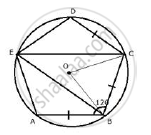Share

Abcde is a Cyclic Pentagon with Centre of Its Circumcircle at Point O Such that Ab = Bc = Cd and Angle Abc = 120° Calculate: (I) ∠Bec (Ii) ∠Bed - ICSE Class 10 - Mathematics

Question

ABCDE is a cyclic pentagon with centre of its circumcircle at point O such that AB = BC = CD and angle ABC = 120°

Calculate:
(i) ∠BEC (ii) ∠BED

Solutioni) Join OC and OB.
AB = BC = CD and ∠ABC  =120°
∴ ∠BCD = ∠ABC = 120°
OB and OC are the bisectors of ∠ABC and ∠BCDrespectively.
∴ ∠OBC = ∠BCO = 60°
In ΔBOC,
∠BOC = 180°  - (∠OBC + ∠BOC)
⇒ ∠BOC =  180° - ( 60° + 60°)
⇒ ∠BOC =  180°  - 120° = 60°
Arc BC subtends ∠BOCat the centre and ∠BEC at the remaining part of the circle.
∴ ∠BEC = 1/2  ∠BOC = 1/2 xx 60° = 30°

ii) In cyclic quadrilateral BCDE,
∠BED +  ∠BCD = 180°
⇒ ∠BED + 120°  =  180°
∴ ∠ BED =  60°

Is there an error in this question or solution?

APPEARS IN

Solution Abcde is a Cyclic Pentagon with Centre of Its Circumcircle at Point O Such that Ab = Bc = Cd and Angle Abc = 120° Calculate: (I) ∠Bec (Ii) ∠Bed Concept: Cyclic Properties.
S